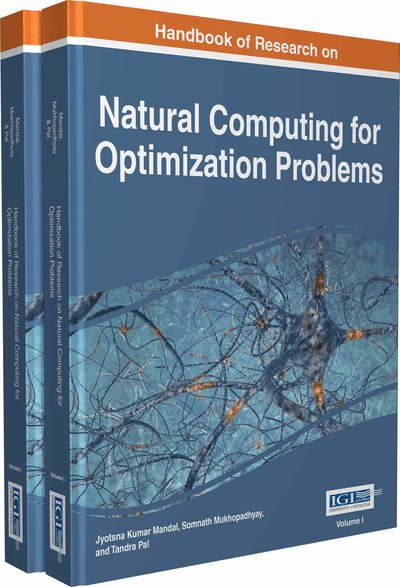# A System on Chip Development of Customizable GA Architecture for Real Parameter Optimization Problem

Sumitra Mukhopadhyay (University of Calcutta, India) and Soumyadip Das (University of Calcutta, India)
DOI: 10.4018/978-1-5225-0058-2.ch004
Available
\$37.50
No Current Special Offers

## Abstract

This chapter presents the design and development of a hardware based architecture of Evolutionary Algorithm for solving both the unimodal and multimodal fixed point real parameter optimization problems. Here a modular architecture has been proposed to provide a tradeoff between real time performance and flexibility and to work as a resource efficient reconfigurable device. The evolutionary algorithm used here is Genetic Algorithm. Prototype implementation of the algorithm has been performed on a system-on-chip field programmable gate array. The notable feature of the architecture is the capability of optimizing a wide class of functions with minimum or no change in the synthesized hardware. The architecture has been tested with ten benchmark problems and it has been observed that for different optimization problems the synthesized target requires maximum of 5% logic slice utilization, 2% of the available block RAMs and 2% of the DSP48 utilization in Xilinx Virtex IV (ML401, XC4VLX25) board.
Chapter Preview
Top

## Introduction

A large number of real world problems like asset allocation, best possible resource utilization, automated system design and operation etc. require decision making process for future evolution of the system under uncertainty. However, in stochastic environment, the problem of decision making involves multiple sub problems like system identification, state estimation and generation of optimal control. Many of these modules require the formulation of mathematical models of the process to be controlled and take aid of several optimization algorithms. Also in various fields of engineering and technology like civil, mechanical, chemical, electronic design automation, VLSI, control, machine learning, signal processing etc. mathematical optimization is applied to find optimal solutions. The optimization problem is generally formulated by representing the different situation of the real world problem in mathematical terms. The possible solutions are represented as the decision variables; the limits of the decision variables are indicative to the range of the solution search space. The objective function is defined, which is usually a function of the decision variables, and its value is required to be optimized (minimized or maximized) to obtain optimum performance of the system satisfying the defined constrains. The unconstrained problems are formulated and optimized without any such constraints.

The standard form of an optimization problem can be defined as follows:

(1)

Subject to

Here, function, f0: RnR is called the cost function or the objective function; the functions fi: RnR, i = 1, . . ., m, are the inequality constraint functions; and, the functions hi: RnR, i = 1, . . ., p, are the equality constraint functions; x = {x1, …,xn} is a vector called the optimization variable of the problem.

A vector z is said to be feasible if it satisfies all the equality and inequality constraints:f1(z)≤0,…., fm(z)≤0;and

h1(z)=0,…., hp(z)=0;

A particular vector x* is called as the optimal solution if it has the optimal objective value among all the feasible vectors:

f0(z)≥ f0(x*);

An optimization problem is said to be unconstrained if m = p = 0.

The task of solving a problem is to find out an acceptable solution, if not be the best one. The search for the better solution is carried out amongst all feasible solutions. The fitness values of the feasible solutions constitute the search space. Thus we search the minimum or maximum point in the search space that represents the better solution to the problem.

Various algorithms (Back, 1996; Holland, 1975; Goldberg, 1989) have been developed for solving the different classes of optimization problems (Boyd & Vandenberghe, 2004). The difficulty of solving the problems depends on the forms and number of constraints, objective functions and variables of the problem. The optimization algorithms can be deterministic or stochastic. Many of the problems require huge computational efforts to find acceptable solutions. As the problem size increases, the solution processes often fail to converge. Bio-inspired stochastic optimization algorithms are developed as effective alternatives to deterministic methods in such a situation for solving optimization problems.

Evolutionary algorithms (EA) optimize a problem by iteratively searching for better solutions utilizing natural phenomena like growth, development, reproduction, selection, survival of the fittest, etc. They include genetic algorithm (GA), differential evolution (DE), and so on.

## Key Terms in this Chapter

Objective Function: It is a real-valued function to be optimized under some constraints and it defines the relationship between input and output of a system which is represented by the function.

Benchmark Problems: It is a set of standard optimization problems consisting of various types of functions, used for evaluation, characterization and performance measurement of optimization algorithm. Behavior of the algorithms under different environmental conditions can be predicted using benchmark functions.

Hardware Description Language: (HDL): A language used to describe the design and functioning of hardware in a software environment that enables to verify the design constraints and then help to implement the design in actual hardware.

Embedded Applications: It is a software or hardware based computer system included as a part of a larger device and dedicated to perform certain real-time function with some constraints.

Evolutionary Algorithm: A set of meta-heuristic, population-based optimization techniques that uses nature inspired processes such as selection, reproduction, recombination, mutation, etc.

Hardware–In-Loop: A platform to test real-time systems consisting of the device under test (DUT) in loop with the mathematical representations of the other related dynamic systems.

System on Chip (SoC): It is a low power computer or an electronic system, capable of various analog and/or digital and/or radio-frequency functions and fabricated into a single integrated circuit (IC).

## Complete Chapter List

Search this Book:
Reset